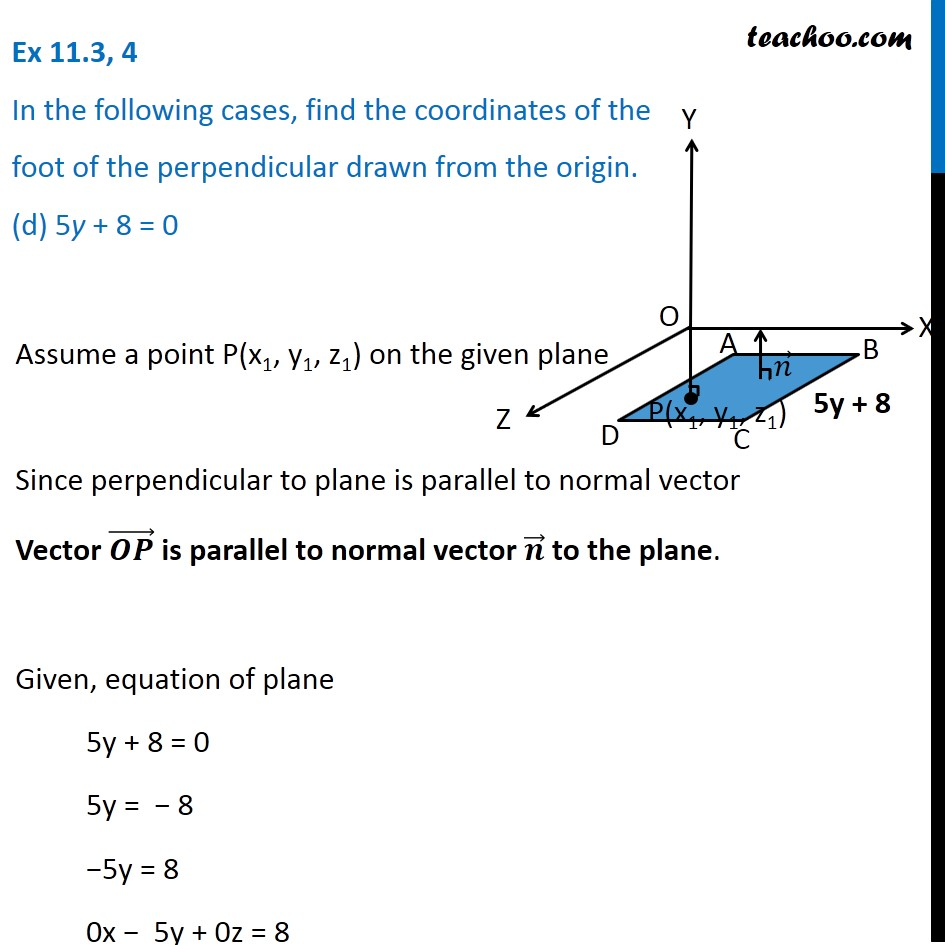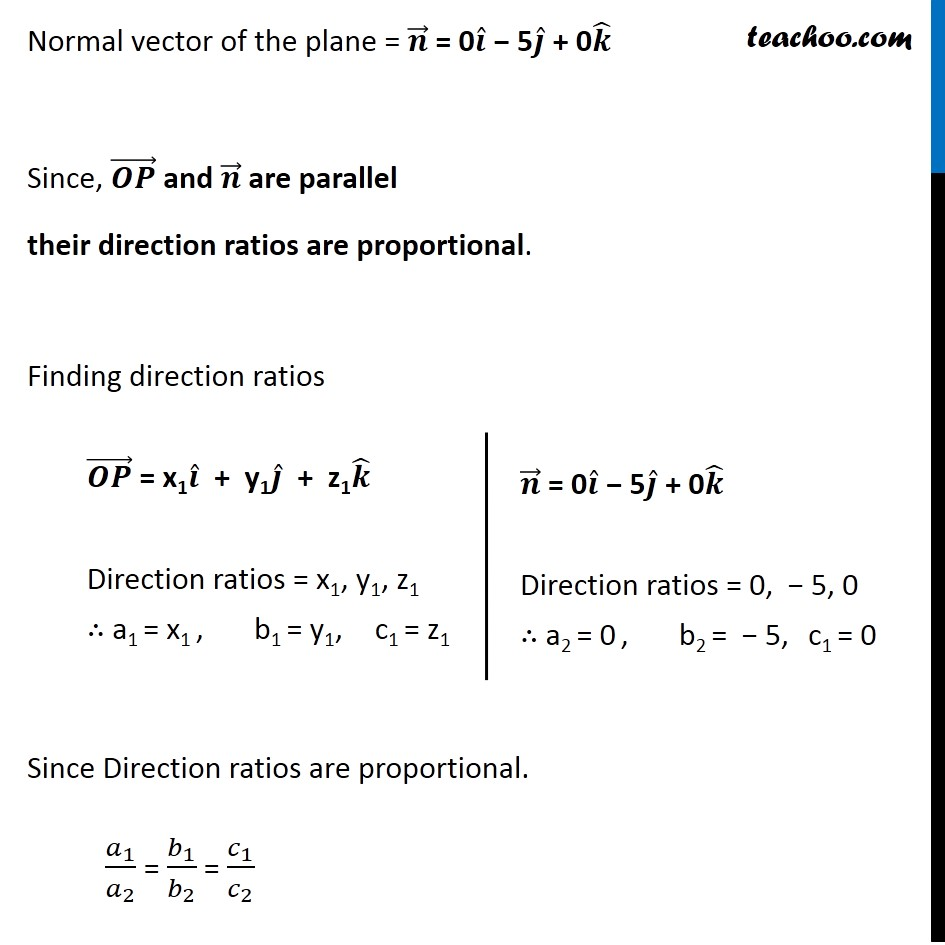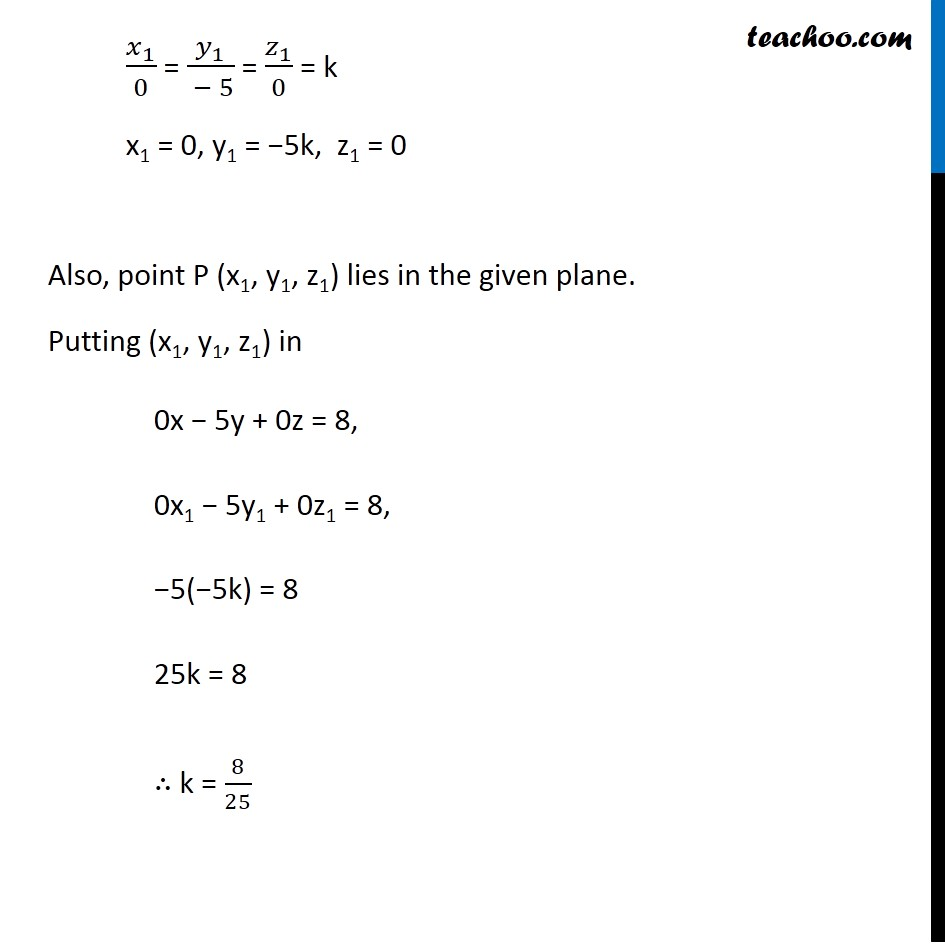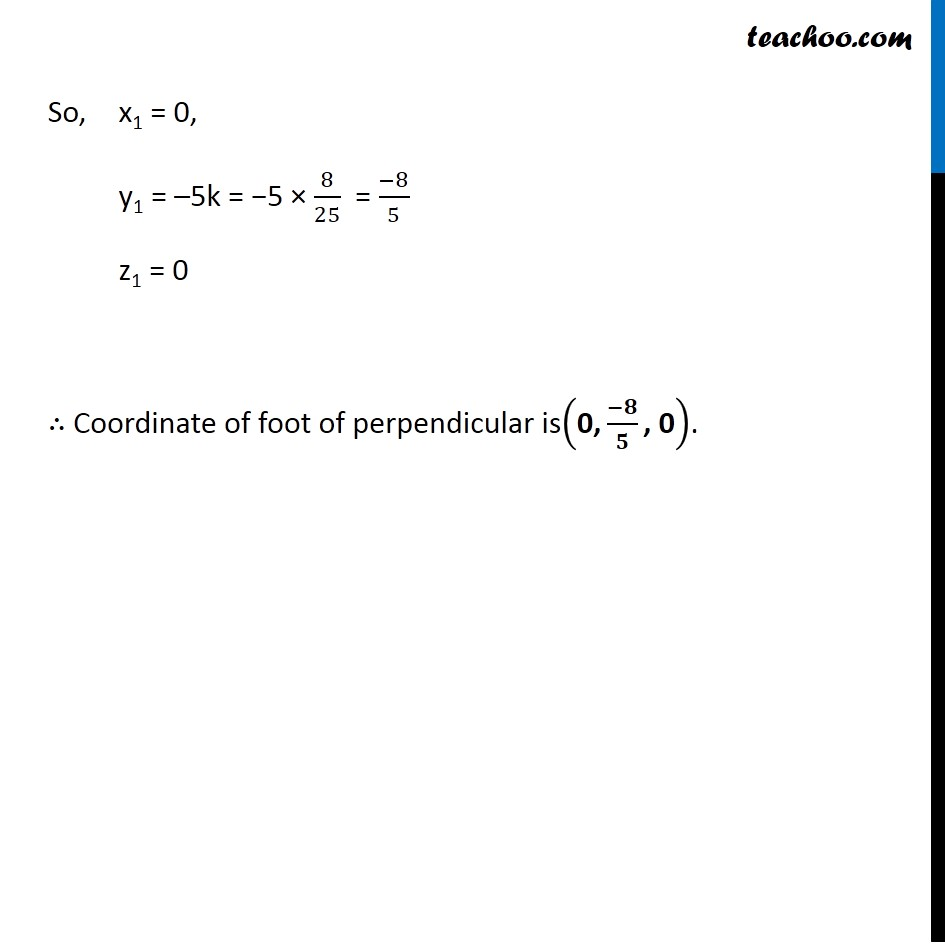Plane

Chapter 11 Class 12 Three Dimensional Geometry
Serial order wiseLearn in your speed, with individual attention - Teachoo Maths 1-on-1 Class

### Transcript

Question 4 In the following cases, find the coordinates of the foot of the perpendicular drawn from the origin. (d) 5y + 8 = 0 Assume a point P(x1, y1, z1) on the given plane Since perpendicular to plane is parallel to normal vector Vector is parallel to normal vector to the plane. Given, equation of plane 5y + 8 = 0 5y = 8 5y = 8 0x 5y + 0z = 8 Since, and are parallel their direction ratios are proportional. Finding direction ratios Since Direction ratios are proportional. 1 2 = 1 2 = 1 2 1 0 = 1 5 = 1 0 = k x1 = 0 , y1 = 5k z1 = 0 Also, point P (x1, y1, z1) lies in the given plane. Putting (x1, y1, z1) in 0x 5y + 0z = 8, 0x1 5y1 + 0z1 = 8, 5( 5k) = 8 25k = 8 k = 8 25 So, x1 = 0, y1 = 5k = 5 8 25 = 8 5 z1 = 0 Coordinate of foot of perpendicular is 0, , 0 .